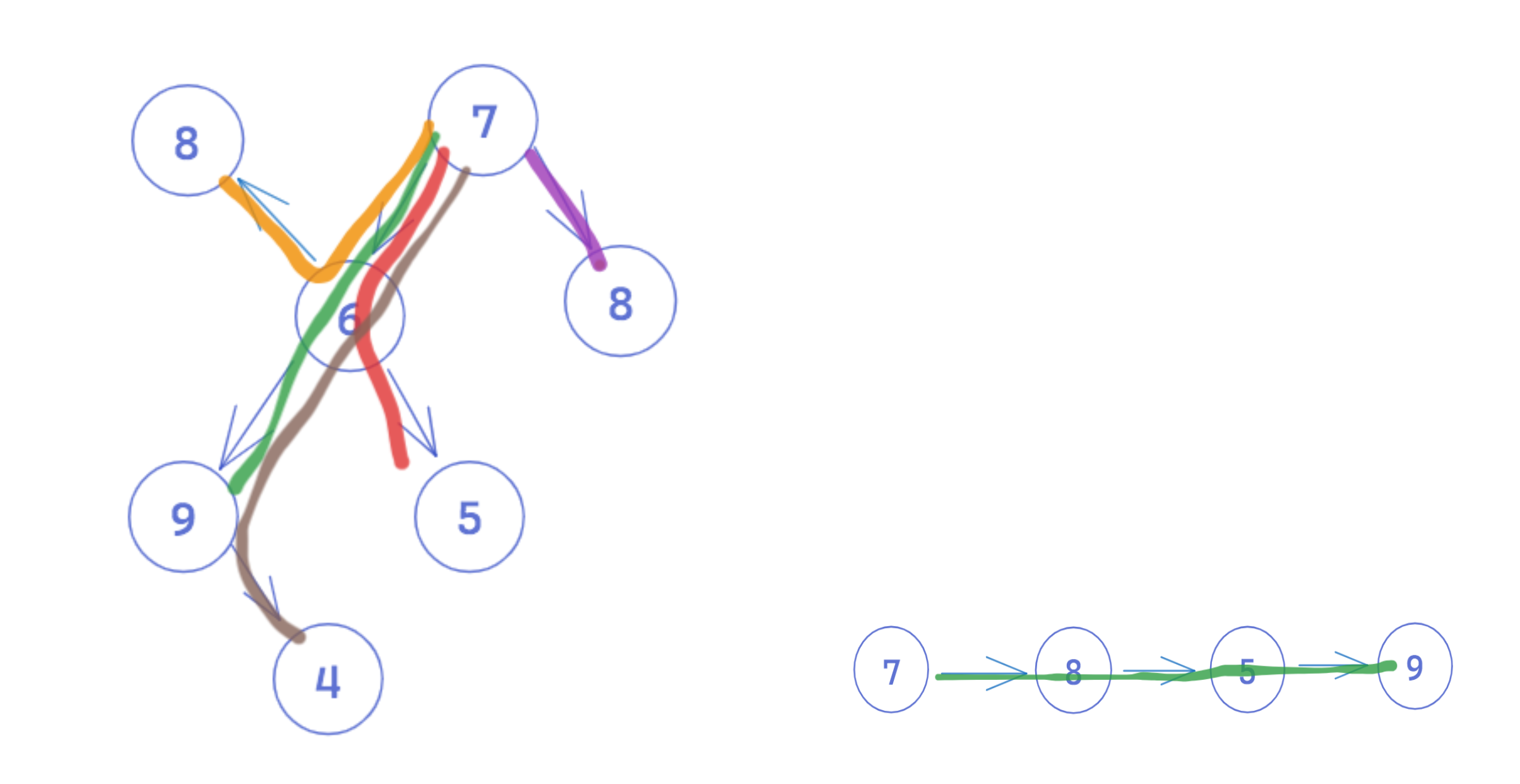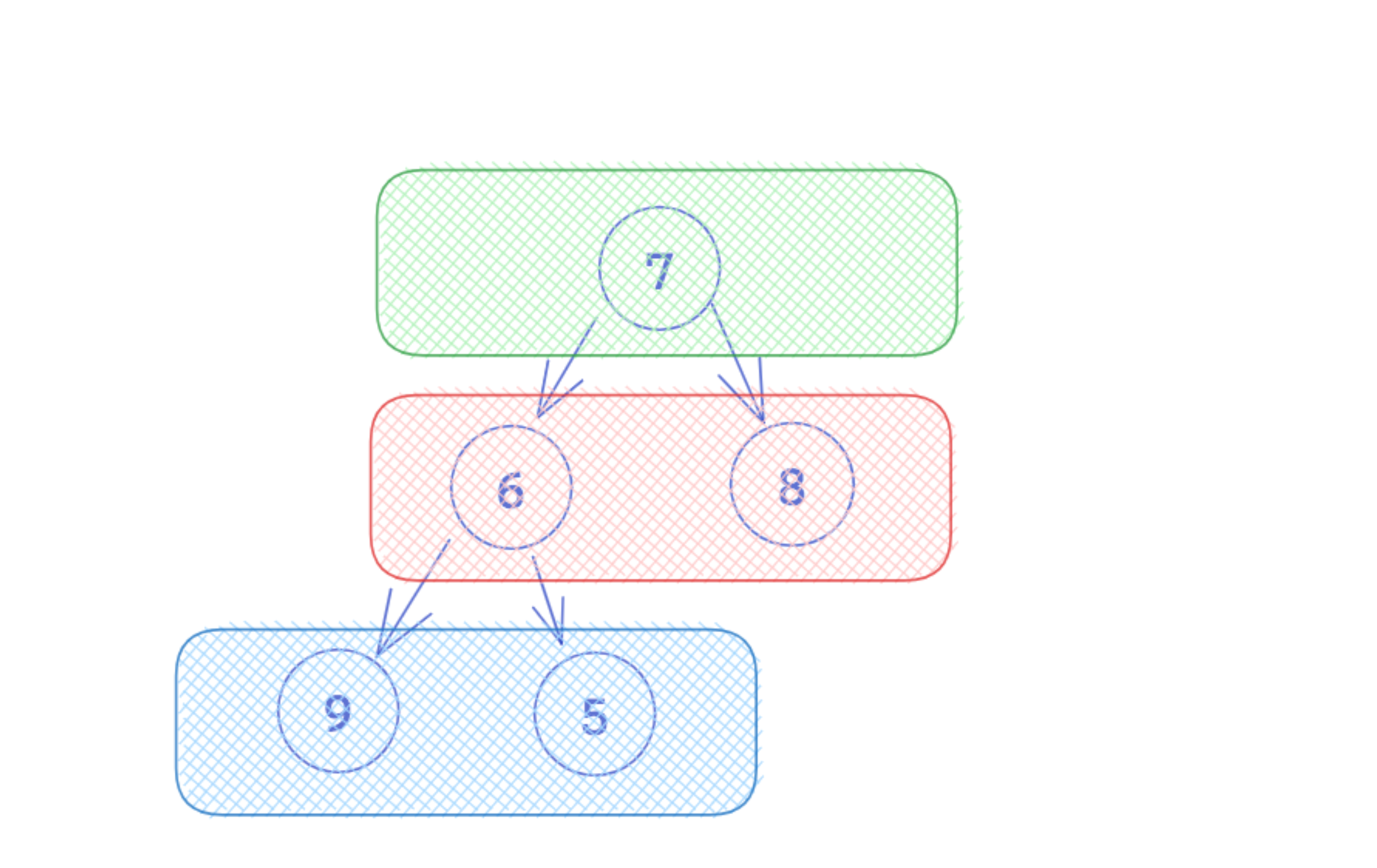By Kenny Polyak and The Mighty Anomaly | Published: July 3, 2023

An essential aspect of working with graphs and trees is understanding how to traverse the search space. Traversal is the process of systematically visiting each node exactly once following a specific order or pattern. This allows us to search for a node or to trace a specific path through the data structure.

Unlike a linear data structure like an array or a linked list - where each node points to only one subsequent node - graphs and trees offer multiple distinct paths to take through the structure. The example below illustrates the many paths that exist through a tree and the single path through a linked list.Different traversal algorithms will produce different traversal orders - knowing which to deploy and when enables us to solve problems more efficiently, and sometimes offers the only way to solve a particular problem. Let's look at how a specific traversal algorithm can be used! At the highest level, there are two main traversal algorithms: Depth-First Search (DFS), which is further distinguished with pre-order, in-order, and post-order traversal when specifically considering DFS in a binary tree, and Breadth-First Search (BFS). In this article, we'll focus on BFS.

Keep in mind that while we can manipulate the traversal path with different algorithms, unless the data structure is ordered in a particular way (like a BST) or our algorithm applies additional logic to omit certain paths, each traversal algorithm will ultimately visit each node once. DFS and BFS are considered blind search algorithms as they do not apply any domain-driven heuristic. Instead, the algorithms only apply traversal rules and a terminal case to determine if a goal state is reached.

Note: Since trees are merely directional, acyclic graphs, we'll just refer to both as graphs in the remainder of this article. Everything discussed below is relevant to trees as well as generic graphs, and in cases where that's not true, it will be pointed out as such.

## What is Breadth-First Search (BFS)?

Breadth-first search (BFS) is an algorithm for traversing tree or graph data structures. Given a node, the algorithm explores all the neighbor nodes first before moving to the next level neighbors, repeating this process until there are no more nodes to visit.

Unlike depth-first search, which traverses as far as possible down a branch as it processes nodes, breadth-first search explores the graph level by level - it's often also referred to as level-order traversal. The result is that all the nodes on a single level are visited at once and grouped together.Starting at the root node, the algorithm visits all the neighbor nodes of a particular node, as well as all the neighbor nodes of the parents at the same level as our current node, before moving to the next level.

## BFS Implementation

BFS typically uses a queue data structure to store the nodes that are waiting to be explored, guaranteeing a FIFO processing order. When a node is visited, all its neighbors are added to the queue in the order they are found. The next node that is visited is grabbed from the front of the queue, whose neighbors are also added to the queue - the queue ensures that nodes are visited in the order they were added. The process continues until all the nodes have been explored or a target state is found.

Here's an overview of the BFS algorithm:

1. Create a visited set to keep track of visited nodes to avoid revisiting them.
2. Create a queue data structure (FIFO) to store the nodes to be processed.
3. Enqueue the starting node onto the queue and mark it as visited.
4. While the queue is not empty, perform the following steps:
• Dequeue a node from the front of the queue.
• Process the dequeued node (e.g., print or perform operations).
• Enqueue all unvisited neighbors of the dequeued node onto the queue and mark them as visited.
5. Repeat step 4 until the queue becomes empty.

Imagine we have a graph with the following node structure:

``````class Node:
def __init__(self, id):
self._id = id
self._neighbors = []
``````

BFS will iteratively add elements to a queue processing all the neighbor nodes until none are left.

``````from collections import deque

class Graph:
def __init__(self):
self.visited = set()

def bfs(self, start_node):
queue = deque([start_node])

# Mark the starting node as visited

while queue:
# Dequeue a node from the front of the queue
current_node = queue.popleft()

# Process the dequeued node
print(current_node.id)

for neighbor in current_node.neighbors:
if neighbor not in self.visited:
# Enqueue unvisited neighbors
queue.append(neighbor)
# Mark the neighbor as visited

# Create Nodes and add neighbors
node1 = Node(1)
node2 = Node(2)
node3 = Node(3)

# Create a Graph object and perform BFS
g = Graph()
g.bfs(node1)
``````

BFS is useful for finding the shortest path between two nodes in a graph or finding the shortest path from a source node to all other nodes in a graph. It is also used for finding all nodes in a graph that are at a certain distance from a given node.

#### Time and Space Complexity

Time and Space Complexity Time complexity: `O(V + E)`, where `V` is the number of vertices and `E` is the number of edges. Space complexity: `O(V)`, where `V` is the number of vertices. This primarily depends on the storage of the visited vertices and the queue used for traversal

#### Time and Space Complexity

Time complexity: `O(V + E)`, where `V` is the number of vertices and `E` is the number of edges, as it must process each vertex and each edge exactly once. Space complexity: `O(V`), where `V` is the number of vertices, as it requires a position in the stack for each vertex.

## When to Use BFS in Technical Interviews

• Finding the shortest distance between two nodes: Problems that require finding the shortest path between nodes are surely meant to test your understanding of BFS. This is often implemented in problems that involve a matrix or a graph that models some physical space, where we are asked to find the shortest distance between two particular cells. BFS prioritizes the nearest nodes first when traversing, which means we can use it to find the shortest path between two nodes, or the minimum number of steps required to reach a goal node or condition.
• When we need to compare a parent to its direct children: Since BFS explores all neighbors of a node before moving on to the next level, this algorithm is useful in scenarios where we want to consider all the children before moving on. This is useful in problems where we need to analyze the relationship between all parents and their direct children.
• When we need to print or analyze data by level in the graph or tree: BFS is also sometimes referred to as "level-order traversal", since we can track all the nodes at a given level. It's useful when we need to batch together all nodes that are at a given level in a tree, or at a given level in a graph relative to some starting node.
• When we want to visit nodes in increasing order of their distance from a start node: Since BFS allows us to track the depth of the algorithm relative to the starting node, it is useful in scenarios where we need to systematically visit nodes at increasing depths from a start node.

## Common Mistakes in Interviews Featuring BFS

• Misunderstanding the tradeoffs and benefits between DFS and BFS: Remember that in many cases both will do just fine, with little to no tradeoffs, but there are specific cases when we should use BFS (finding the shortest distance between two nodes, for example).
• Not using the correct supporting data structure: BFS is made possible with a queue data structure, while DFS relies on a stack. It's important to be able to identify this when implementing, and to be sure not to use the wrong supporting data structure.
• Forgetting to track visited nodes: When dealing with a graph that is not guaranteed to be directed and acyclic, it is essential to track visited nodes in order to avoid an infinite loop. Interviewers will be looking for this as a common pitfall. One way to ensure you don't forget is to perform a manual dry-run of your algorithm.
• Choosing BFS over DFS when it's not needed: Although in many cases these are both interchangeable, one big benefit of using DFS for backtracking algorithms is that memoization can be implemented easily.

## Common BFS interview QuestionsKenny Polyak

Kenny is a software engineer and technical leader with four years of professional experience spanning Amazon, Wayfair, and U.S. Digital Response. He has taught courses on Data Structures and Algorithms at Galvanize, helping over 30 students land new software engineering roles across the industry, and has personally received offers from Google, Square, and TikTok.The Mighty Anomaly

The Mighty Anomaly (Mike) is one of the top Google coaches for interviewing.io having personally helped over 100+ engineers get into Google with his unique coaching style. After receiving offers from the full FAANG gauntlet early in his career, he enjoys teaching others proven techniques to pass technical interviews with his decade of coaching and industry experience.